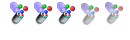## MCAT practice questions

MCAT preparation and implications of MCAT scores.

Moderators: cheechootrain, CaribMD, AL_emt

### MCAT practice questions

A student throws a rock at a 60° angle over a lake. The rock leaves his hand at a velocity of 20 m/s. How far away from the student will the rock shadow appear on the ground after 1 second, assuming the shadow projects straight down from the rock?
A) 5 m
B) 7 m
C) 10 m
D) 12 m

You can find more free MCAT questions here https://www.mcat-prep.com/mcat-practice ... ctice-Test
Last edited by goldstandard_mcat on Mon Jul 18, 2016 6:04 am, edited 1 time in total.
goldstandard_mcat
GunnerPosts: 59
Joined: Thu May 15, 2014 2:49 am

### Re: MCAT practice questions

The real answer would be "insufficient information", because the question does not take into account various physical factors such as wind speed and direction, atmospheric pressure, whether the student is above/below/at the same level as the lake. etc
Percival B
GunnerPosts: 72
Joined: Wed Aug 14, 2013 5:58 pm

### Re: MCAT practice questions

Answer is C. Because the question asks for the distance between the rock’s shadow and the student, the distance will be a horizontal distance. The horizontal velocity of the rock is given by vx= v•cosθ= v•cos60o= v (1/2). Thus vx=10 m/s. So, distance x is given by x= (vx)(t)= 10 m/s (1s)= 10 m.

goldstandard_mcat wrote:A student throws a rock at a 60° angle over a lake. The rock leaves his hand at a velocity of 20 m/s. How far away from the student will the rock shadow appear on the ground after 1 second, assuming the shadow projects straight down from the rock?
A) 5 m
B) 7 m
C) 10 m
D) 12 m
goldstandard_mcat
GunnerPosts: 59
Joined: Thu May 15, 2014 2:49 am

### Re: MCAT practice questions

Which of the following typically have a plasma membrane but no nuclear membrane?
A) Bacteria
B) Bacteriophages
C) Fungi
D) Viruses other than bacteriophages
goldstandard_mcat
GunnerPosts: 59
Joined: Thu May 15, 2014 2:49 am

### Re: MCAT practice questions

Incorrect.

For example: if the lake is at a different level then the shadow will be a diagonal distance from the student.

You just copied that incorrect answer from a commercial-prep MCAT book or simplistic textbook.
Lots of scientific reasons why you are wrong, and why the question would not be on a real MCAT.

The answer is: your laziness, a poor example of a physics multiple-choice problem, and this calls into question whether you have ever taken a course in the physical sciences.
Percival B
GunnerPosts: 72
Joined: Wed Aug 14, 2013 5:58 pm

Next